﻿ 层面接触刚度对叠合岩梁应力分布的影响规律

层面接触刚度对叠合岩梁应力分布的影响规律Influence Law of Level Contact Stiffness on Stress Distribution of Laminated Rock Beam

Abstract: In order to investigate the influence of contact stiffness parameters on the stress distribution of laminated beams, a numerical model of double deck laminated beams under different contact stiffness parameters is established by FLAC3D, and the influence of shear stiffness Ks and normal stiffness Kn on the distribution of stress distribution on both ends of the laminated beam under uniform load conditions is analyzed. The results show that the stress distribution of the double deck fixed rock beam under uniform load is obviously related to the contact stiffness of the layer. When the shear stiffness Ks increases, the maximum tensile stress and the maximum compressive stress in the horizontal direction of the rock beam decrease, and the tensile stress in the horizontal direction increases gradually with the normal stiffness Kn, and the compressive stress gradually decreases. At the same time, when Kn and Ks increase to a certain value, the stress distribution of rock beam changes slightly with the change of contact stiffness, and tends to be stable. Through the research in this paper, it can provide some reference for selecting reasonable contact stiffness parameters in numerical calculation.

1. 引言

2. 层面的变形性质

2.2. 法向变形性质

$u={u}_{0}\left(1-{\text{e}}^{-\frac{{\sigma }_{n}}{{K}_{n}}}\right)$ (1)

${K}_{n}=\frac{\partial {\sigma }_{n}}{\partial \Delta {V}_{j}}$ (2)

2.2. 剪切变形性质

${K}_{s}=\frac{\partial \tau }{\partial {u}_{s}}$ (3)

${\tau }_{n}={\sigma }_{v}tg\varphi +C$ (4)

3. 数值模型的建立

3.1. 模型概述

3.2. 参数的选取

3.3. 监测点的布置

Flac3d模拟中把模型分为240个单元体，监测单元体应力和位移，选取A、B两个单元体作为监测点，监测其水平方向应力和垂直方向应力，监测A和B单元体垂向位移。A单元体坐标为(x, y, z)，(x = 15, 16; y = 0, 1; z = 2, 3)，B单元体坐标为(x, y, z)，(x = 15, 16; y = 0, 1; z = 6, 7)，A、B单元体在叠合岩梁模型中位置如图2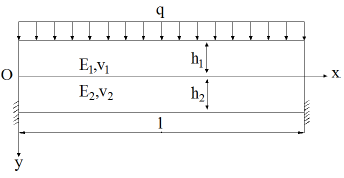Figure 1. Analysis model of laminated rock beamTable 1. Parameter value table for analysis model of laminated rock beam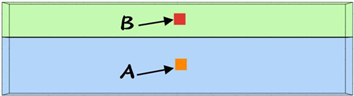Figure 2. Location of monitoring points A and B

4. 模拟结果分析

4.1. 应力分析

1) 改变剪切刚度

2) 改变法向刚度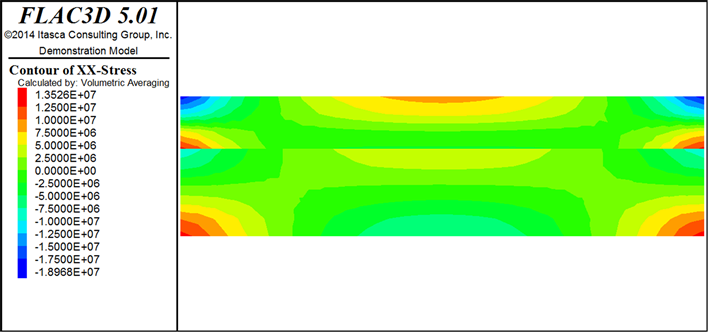(a)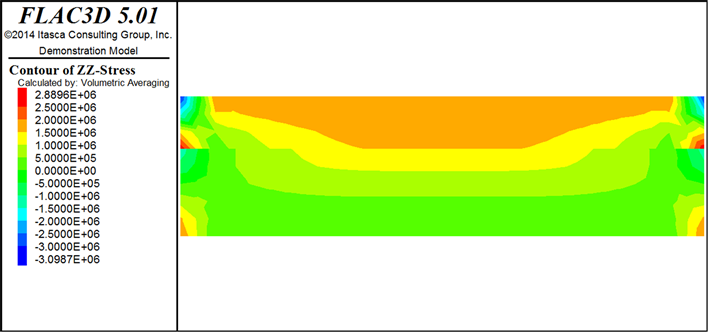(b)

Figure 3. Stress distribution of laminated rock beams(a)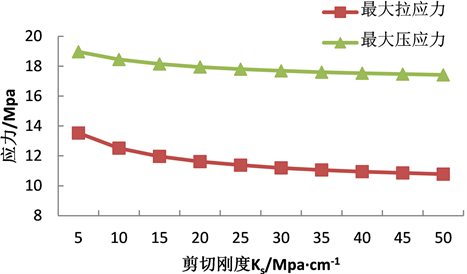(b)

Figure 4. Maximum stress change of laminated rock beam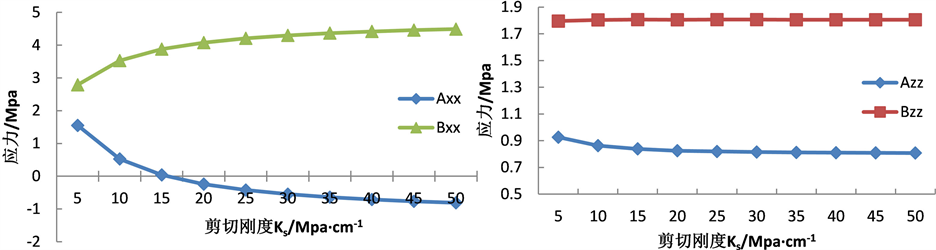(a) (b)

Figure 5. Stress change of cell body A and B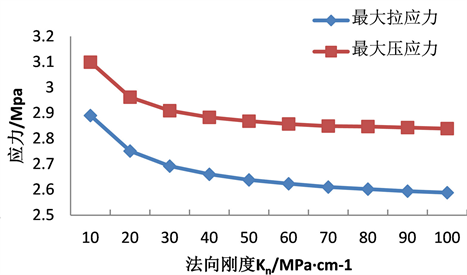(a)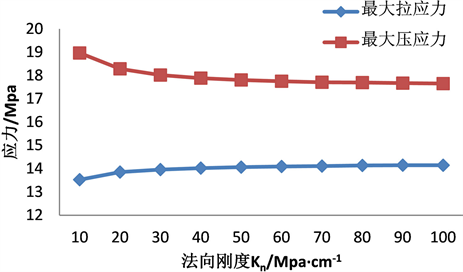(b)

Figure 6. Maximum stress change of laminated rock beam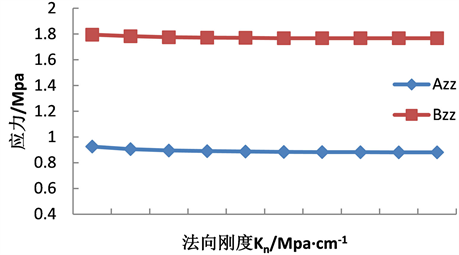(a)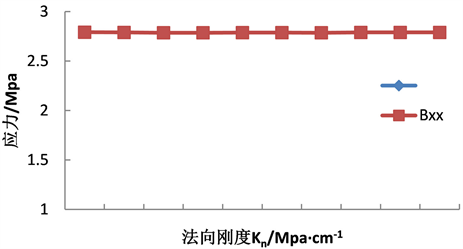(b)

Figure 7. Stress change of cell body A and B

4.2. 位移分析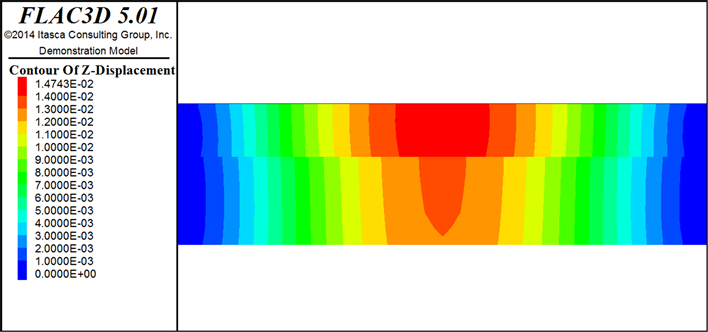Figure 8. Vertical displacement of laminated rock beams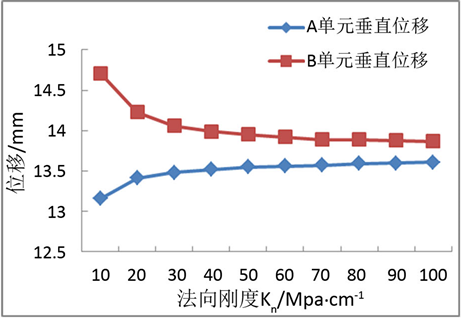(a)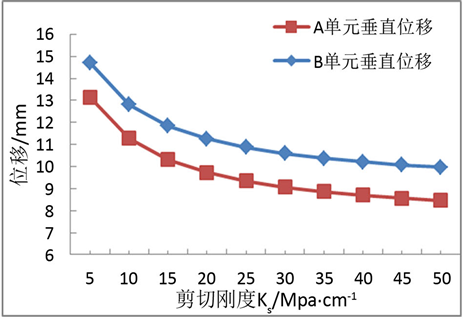(b)

Figure 9. Vertical displacement of element A and B

A单元位于叠合岩梁下层，B单元位于岩梁上层，随着层面接触刚度的变化，在上覆均匀荷载作用下，岩梁垂直方向位移有较大变化，其水平方向位移忽略不计。

5. 结论

a) 双层叠合简支岩梁在上覆均匀荷载作用下，内部应力分布受中间层面接触刚度的影响，上层应力大于下层应力，上层应力通过接触面时减弱。层面变形性质包括层面的剪切刚度Ks和法向刚度Kn，二者的变化对于双层叠合岩梁内部应力分布有着很大的影响。

b) 当增加接触面剪切刚度Ks时，岩梁水平方向最大拉应力和最大压应力都减小，岩梁上、下层最大应力与剪切刚度Ks呈负相关；当剪切刚度Ks小于15 Mpa/cm时，岩梁垂直方向的最大应力随着Ks的增大而减小，反之则不变。随着剪切刚度Ks的增大，岩梁下层内部的水平和垂直方向应力减小，上层内部的应力增大。当Ks大于15 Mpa/cm时，岩梁内部应力变化趋势变缓直至趋于稳定值。

c) 当增加接触面法向刚度Kn时，叠合岩梁水平方向最大拉应力逐渐增加并趋于稳定达到14.15 Mpa，最大压应力逐渐减小到17.65 Mpa；其垂直方向最大拉应力和压应力均减小，当Kn大于30 Mpa/cm时，减速变缓。岩梁内部上、下层的水平和垂直方向应力发生轻微变化，当Kn小于30 Mpa/cm时，岩梁下层的水平应力增大，垂直应力减小，反之则基本不变。岩梁上层内部应力基本不变。

d) 岩梁垂直位移由跨中向两端均匀减小。由于上、下层接触面接触刚度的作用，距垮中等距时上层位移大于下层位移。当增加剪切刚度Ks时，岩梁上下层垂直位移都减小，下层位移小于上层。当增加法向刚度Kn，岩梁下层垂直位移增大，上层垂直位移减小，A单元位移小于B单元。当Kn大于30 Mpa/cm时，下层和上层垂直位移增速和减速变缓并趋于稳定。

 贾蓬, 唐春安, 王述红. 巷道层状岩层顶板破坏机理[J]. 煤炭学报, 2006, 31(1): 11-15.

 李德海. 近水平层状岩层移动规律的探究[J]. 采矿与安全工程学报, 1996(2): 39-42.

 卜庆为, 高阳, 常瑞. 水平应力作用下层状顶板回采巷道围岩失稳机理及支护分析[J]. 煤炭技术, 2017, 36(11): 12-14.

 王洪建, 刘大安, 黄志全, 等. 层状页岩岩石力学特性及其脆性评价[J]. 工程地质学报, 2017, 25(6): 1414-1423.

 徐嘉谟, 马凤山, 曹家源, 等. 岩体在工程状态下间断面的力学效应[J]. 工程地质学报, 2018, 26(1): 10-23.

 梁运培, 孙东玲. 岩层移动的组合岩梁理论及其应用研究[J]. 岩石力学与工程学报, 2002(5): 654-657.

 黄菊华, 钱应平, 李厚民. 不同形式叠合梁的应力分析[J]. 湖北工业大学学报, 2008(3): 87-89.

 徐铎, 赵建峰, 田慧强, 等. 横向叠加岩梁截面应力分析[J]. 煤炭工程, 2011(10): 113-116.

 孟召平, 陆鹏庆, 贺小黑. 沉积结构面及其对岩体力学性质的影响[J]. 煤田地质与勘探, 2009, 37(1): 33-37.

 孙宗颀. 不连续面应力–变形性质的研究[J]. 岩石力学与工程学报, 1987(4): 287-300.

 刘佑荣, 唐辉明. 岩体力学[M]. 武汉: 中国地质大学出版社, 1999.

 孙广忠. 岩体结构力学[M]. 北京: 科学出版社, 1988.

 潭学术. 复合岩体力学理论及其应用[M]. 北京: 煤炭工业出版社, 1994.

 苗丹, 刘一华. 双材料叠合简支梁受均布载荷作用时的解析解[J]. 应用力学学报, 2016, 33(4): 589-595, 735.

Top